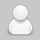# 十分钟搞定时间复杂度（算法的时间复杂度）

```int aFunc(void) {
printf("Hello, World!\n");    //  需要执行 1 次
return 0;              // 需要执行 1 次
}```

```int aFunc(int n) {
for(int i = 0; i<n; i++) {    // 需要执行 (n + 1) 次
printf("Hello, World!\n");     // 需要执行 n 次
}
return 0;               // 需要执行 1 次
}```1、我们知道常数项对函数的增长速度影响并不大，所以当 T(n) = c，c 为一个常数的时候，我们说这个算法的时间复杂度为 O(1)；如果 T(n) 不等于一个常数项时，直接将常数项省略。

```比如

T(n) = n + 29，此时时间复杂度为 O(n)。```

2、我们知道高次项对于函数的增长速度的影响是最大的。n^3 的增长速度是远超 n^2 的，同时 n^2 的增长速度是远超 n 的。 同时因为要求的精度不高，所以我们直接忽略低此项。

```比如
T(n) = n^3 + n^2 + 29，此时时间复杂度为 O(n^3)。```

3、因为函数的阶数对函数的增长速度的影响是最显著的，所以我们忽略与最高阶相乘的常数。

```比如
T(n) = 3n^3，此时时间复杂度为 O(n^3)。```

1、对于一个循环，假设循环体的时间复杂度为 O(n)，循环次数为 m，则这个循环的时间复杂度为 O(n×m)。

```void aFunc(int n) {
for(int i = 0; i < n; i++) {    // 循环次数为 n
printf("Hello, World!\n");      // 循环体时间复杂度为 O(1)
}
}```

2、对于多个循环，假设循环体的时间复杂度为 O(n)，各个循环的循环次数分别是a, b, c...，则这个循环的时间复杂度为 O(n×a×b×c...)。分析的时候应该由里向外分析这些循环。

```void aFunc(int n) {
for(int i = 0; i < n; i++) {       // 循环次数为 n
for(int j = 0; j < n; j++) {     // 循环次数为 n
printf("Hello, World!\n");         // 循环体时间复杂度为 O(1)
}
}
}```

3、对于顺序执行的语句或者算法，总的时间复杂度等于其中最大的时间复杂度。

```void aFunc(int n) {
// 第一部分时间复杂度为 O(n^2)
for(int i = 0; i < n; i++) {
for(int j = 0; j < n; j++) {
printf("Hello, World!\n");
}
}
// 第二部分时间复杂度为 O(n)
for(int j = 0; j < n; j++) {
printf("Hello, World!\n");
}
}```

4、对于条件判断语句，总的时间复杂度等于其中 时间复杂度最大的路径 的时间复杂度。

```void aFunc(int n) {
if (n >= 0) {
// 第一条路径时间复杂度为 O(n^2)
for(int i = 0; i < n; i++) {
for(int j = 0; j < n; j++) {
printf("输入数据大于等于零\n");
}
}
} else {
// 第二条路径时间复杂度为 O(n)
for(int j = 0; j < n; j++) {
printf("输入数据小于零\n");
}
}
}```

```void aFunc(int n) {
for (int i = 0; i < n; i++) {
for (int j = i; j < n; j++) {
printf("Hello World\n");
}
}
}```

```void aFunc(int n) {
for (int i = 2; i < n; i++) {
i *= 2;
printf("%i\n", i);
}
}```

```long aFunc(int n) {
if (n <= 1) {
return 1;
} else {
return aFunc(n - 1) + aFunc(n - 2);
}
}```

### 解决Chrome开启硬件加速后播放视频黑屏闪烁

#### 发表评论◎欢迎参与讨论，请在这里发表您的看法和观点。